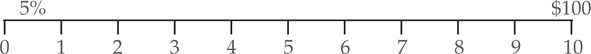# Present Value of a Sum

Sometimes, we want to define what the value is today of sums that we’ll receive in the future. For example, if a firm is evaluating an investment that will generate future income, it must compare today’s expenditures with expected future revenues. In order to compare sums across time, future values must be adjusted to what they’re worth today.

Present Value Formula

Take another look at the future value formula:

$F{{V}_{n}}=P{{V}_{o}}{{\left( 1+i \right)}^{n}}$

The future value is defined in terms of present value. To find the equation for computing present value, we need to rearrange the above formula. If both sides are divided by (1+i)n, we get the equation for present value:

$\begin{matrix}P{{V}_{0}}=\frac{F{{V}_{n}}}{{{\left( 1+i \right)}^{n}}}=F{{V}_{n}}{{\left( 1+i \right)}^{-n}} & {} & \left( 1 \right) \\\end{matrix}$

The term 1/(1+i)n (or (1+i)−n) is called the present value interest factor.

Example 1 Solving for the Present Value

What is the present value of \$100, which will be received in 10 years, if the interest rate is 5%?

Solution

\begin{align}& FV=\100\\&n=10\\&i=0.05\\\end{align}\begin{align}& P{{V}_{0}}=F{{V}_{n}}\times \frac{1}{{{\left( 1+i \right)}^{n}}} \\& =\100\times\frac{1}{{{\left(1.05\right)}^{10}}}=\100\times0.61391=\61.39\\\end{align}